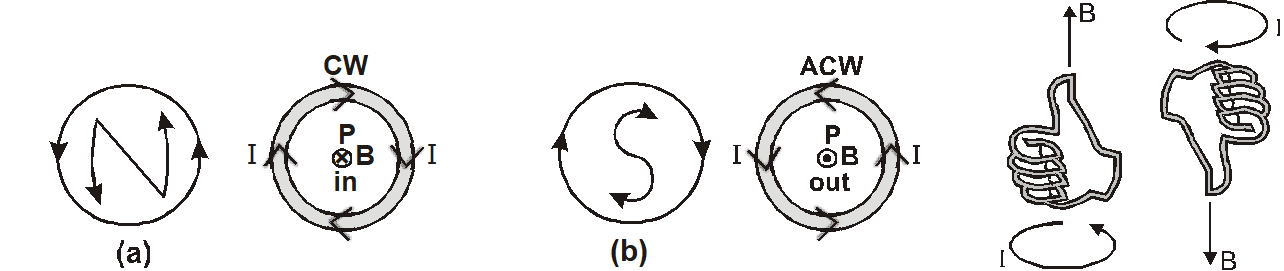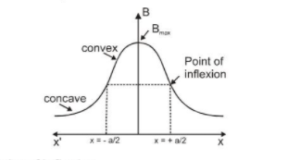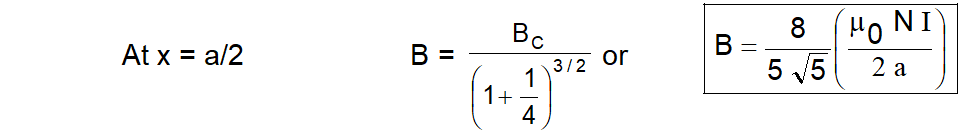Most Affordable JEE | NEET | 8,9,10 Preparation by Kota's Top IITian Doctor Faculties

# Right Hand Palm Rule - Magnetic Effect of Current Class12, JEE & NEETSo far we have described the magnitude of the magnetic force on a moving electric charge, but not the direction. The magnetic field is
a vector field, thus the force applied will be oriented in a particular direction. There is a clever way to determine this direction using nothing more than your right hand. The direction of the magnetic force $F$ is perpendicular to the plane formed by $v$ and $B$, as determined by the right-hand palm rule, which is illustrated in the figure. The right-hand rule states that to determine the direction of the magnetic force on a positive moving charge, $f$, point the thumb of the right hand in the direction of $v,$ the fingers in the direction of $B,$ and a perpendicular to the palm points in the direction of $F$

## Right Hand Palm Rule

If we hold the thumb of the right hand mutually perpendicular to the grip of the fingers such that the curvature of the finger represents the direction of current in the wire loop, then the thumb of the right hand will point in the direction of the magnetic field near the center of the current loop### Graph of B v/s X

As soon as x increases magnetic field B decreases, dependence of B on x is shown in figure

Rate of change of B with respect to x is different at different values of x

for $x<\pm \frac{a}{2}$ curve is convex and $\quad$ for $x>\pm \frac{a}{2}$ curve is concave

At $x=\pm \frac{a}{2} \quad$ we get $\frac{d B}{d x}=$ const $,$ and $\frac{d^{2} B}{d x^{2}}=0$

So at $x=+\frac{a}{2} \&-\frac{a}{2}$ B varies linearly with $x$These points are called points of inflexion.

Distance in between these two points is equal to radius of the coil

$B=\frac{B_{C}}{\left(1+\frac{x^{2}}{a^{2}}\right)^{3 / 2}}$

$\because$ Magnetic field at the centre of coil $\mathrm{B}_{\mathrm{C}}=\frac{\mu_{0} \mathrm{NI}}{2 \mathrm{a}}$Biot Savart's Law
Click here for the Video tutorials of Magnetic Effect of Current Class 12
About eSaral At eSaral we are offering a complete platform for IIT-JEE & NEET preparation. The main mission behind eSaral is to provide education to each and every student in India by eliminating the Geographic and Economic factors, as a nation’s progress and development depends on the availability of quality education to each and every one. With the blend of education & technology, eSaral team made the learning personalized & adaptive for everyone.